## 我有礼品你有故事吗 —— Java 开发规约勾起了你什么样的回忆？ 热

ON! HashMap 的并发操作导致死循环了！1、说说自己因为里面的某些条款踩过的坑，我们将设置 10 个“最悲催同学奖”；

2、针对里面的条款找找茬，说说里面有哪些条款不合适，我们将设置 10 个“最佳评论奖”；int ONE=1;
int TWO=2;
int THREE =3;
......
int TEN=10;

……3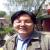p

p

1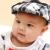1.这坑就太多了，旁边一个哥们提交的代码给你们瞅瞅

``````        List<Map<String, Object>> mapList = new ArrayList<Map<String, Object>>();
List<Map<String, Object>> mapList1 = new ArrayList<Map<String, Object>>();
Integer hiddConred;
Integer hiddConh;
Integer hiddConl;

Integer c = 0;
List<Dept> deptList = deptService.queryDeptByPid(1);

/* 获取情报分类列表 */
List<AstDictionaries> listf = astDictionariesService.reclassification();
for (AstDictionaries astDictionaries : listf) {
Map<String, Object> map = new HashMap<String, Object>();
c = c + 1;
Integer sd = polIntelligenceService.huodefenlei(astDictionaries.getId());
map.put("a" + c, sd);

}``````

2.记得第二年工作的时候经理给过一份类似阿里这样的代码规范，从此之后代码就美观多了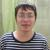p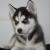1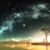java编程从业也有几年了，回想多年前刚开始接触java的时候真是感慨良多呀！

1.了解了定义变量，如String a="i love you",自学了集合类的时候Set<?> x=HashSet<>()，编译错误居然没有发现忘记了"new";

2.非常喜欢用Integer i=128，来刁难别人。

3.心烦的时候思考逻辑真的是一团糟呀一团糟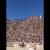1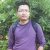1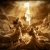Integer i1=1;

Integer i2=1;

Integer i3=127;

Integer i4=127;

Integer i5=128;

Integer i6=128;

Integer i7=-128;

Integer i8=-128;

i1==i2 返回true

i3==i4 返回true

i4==i6 返回true

i7==i8 返回true

1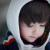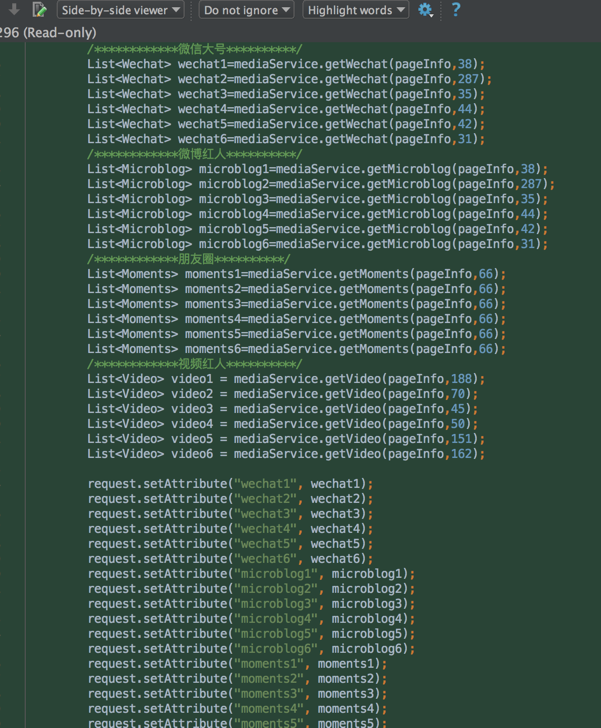0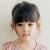``````boolean a=true;

boolean b=false;

while(a=b){

....

}``````